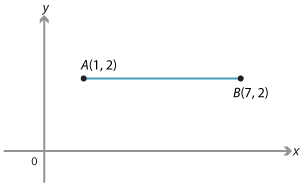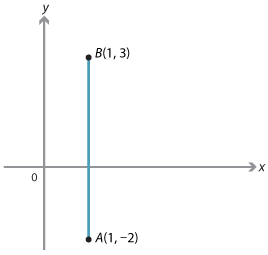### The midpoint of an interval

The coordinates of the midpoint of a line interval can be found using averages as we will see.

We first deal with the situation where the points are horizontally or vertically aligned.

#### Example 1

Find the coordinates of the midpoint of the line interval AB, given the following points:

1. $$A(1, 2)$$ and $$B(7, 2)$$
2. $$A(1, −2)$$ and $$B(1, 3)$$

#### Solution

1. AB is a horizontal line interval, the midpoint is at (4, 2), since 4 is halfway between 1 and 7.Note that 4 is the average of 1 and 7, that is: 4 = $$\dfrac{1 + 7}{2}$$.
2. The midpoint of AB has coordinates $$(1, \dfrac{1}{2})$$.Note that $$\dfrac{1}{2}$$ is the average of 3 and $$-2$$.